# mne.convert_forward_solution#

mne.convert_forward_solution(fwd, surf_ori=False, force_fixed=False, copy=True, use_cps=True, *, verbose=None)[source]#

Convert forward solution between different source orientations.

Parameters:
fwd`Forward`

The forward solution to modify.

surf_ori`bool`, optional (default `False`)

Use surface-based source coordinate system? Note that force_fixed=True implies surf_ori=True.

force_fixed`bool`, optional (default `False`)

If True, force fixed source orientation mode.

copy`bool`

Whether to return a new instance or modify in place.

use_cps`bool`

Whether to use cortical patch statistics to define normal orientations for surfaces (default True).

verbose

Control verbosity of the logging output. If `None`, use the default verbosity level. See the logging documentation and `mne.verbose()` for details. Should only be passed as a keyword argument.

Returns:
fwd`Forward`

The modified forward solution.

## Examples using `mne.convert_forward_solution`#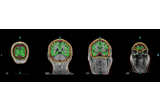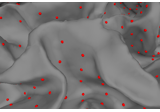The role of dipole orientations in distributed source localization

The role of dipole orientations in distributed source localization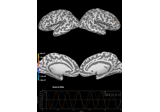Corrupt known signal with point spread

Corrupt known signal with point spread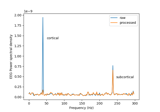Cortical Signal Suppression (CSS) for removal of cortical signals

Cortical Signal Suppression (CSS) for removal of cortical signals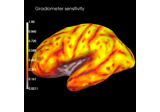Display sensitivity maps for EEG and MEG sensors

Display sensitivity maps for EEG and MEG sensors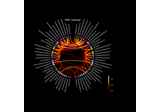Visualize source leakage among labels using a circular graph

Visualize source leakage among labels using a circular graph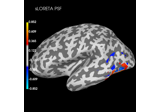Plot point-spread functions (PSFs) and cross-talk functions (CTFs)

Plot point-spread functions (PSFs) and cross-talk functions (CTFs)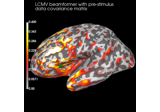Compute cross-talk functions for LCMV beamformers

Compute cross-talk functions for LCMV beamformers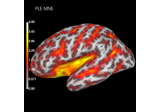Compute spatial resolution metrics in source space

Compute spatial resolution metrics in source space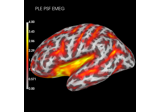Compute spatial resolution metrics to compare MEG with EEG+MEG

Compute spatial resolution metrics to compare MEG with EEG+MEG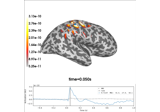Optically pumped magnetometer (OPM) data

Optically pumped magnetometer (OPM) data